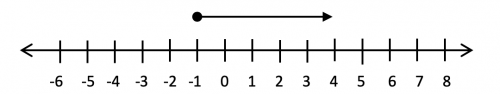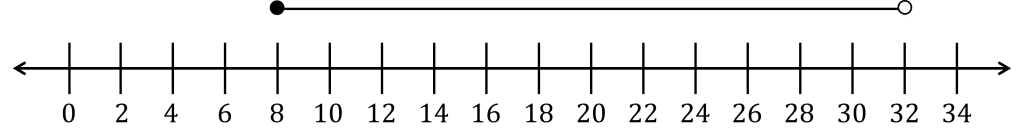# Inequalities On a Number Line

GCSEKS3Level 4-5AQAEdexcelEdexcel iGCSEOCRWJEC

## Inequalities

The equations we are most familiar with are equalities, where the left hand side and the right hand side are identical or equal. It is also useful to have a way of expressing a range of values instead of a single specific value which can be achieved by using inequalities. A common way to display inequalities is using a number line.

Level 4-5GCSEKS3AQAEdexcelOCRWJECEdexcel iGCSE

## Inequality Symbols and their Meaning

Before learning about how to display inequalities on a number line, you firstly need to know the following symbols and their definitions:

• $>$ means “greater than”,
• $\geq$ means “greater than or equal to”,
• $<$ means “less than”,
• $\leq$ means “less than or equal to”.

We call $\leq$ and $\geq$ inclusive inequalities, and we call $<$ and $>$ strict inequalities. For example $x\leq 8$ includes the value $8$ as a possibility for $x$, whilst the inequality $x<8$ does not.

Level 4-5GCSEKS3AQAEdexcelOCRWJECEdexcel iGCSE

## Type 1: Inequalities on a Number Line

The inequality, $x\geq -5$ means that $x$ can take any value that is bigger than $-5$, including $-5$.

We can display this on a number line by drawing a filled in circle at $-5$ and an arrow pointing to the right hand side indicating the numbers that are greater than $-5$. This should look like,When drawing inequalities on a number line it is important to remember that you should use an closed circle, $\bullet$, for an inclusive inequality, e.g. $x\geq-5$ and use a open circle, $\circ$, for a strict inequality, e.g. $x\lt2$.

Level 4-5GCSEKS3AQAEdexcelOCRWJECEdexcel iGCSE

## Type 2: Inequalities on a Number Line

Inequalities can describe a range of values between an upper and lower limit. For example the inequality $-3 \leq x \lt 5$ means that $x$ can take any value greater than or equal to $-3$ but also has to be less than $5$.

Here, the first part of the inequality is an inclusive inequality so is drawn with a filled in circle and the second part is a strict inequality so is drawn with a empty circle.

Example: Draw $-3 \leq x \lt 5$ on the number line below.Level 4-5GCSEKS3AQAEdexcelOCRWJECEdexcel iGCSE
Level 4-5GCSEKS3AQAEdexcelOCRWJECEdexcel iGCSE

## Example 1

Show the inequality $-1 \lt x \lt 10$ on a number line.

[2 marks]

Key points when drawing the number line.

• The circles indicate the limits of the inequality ($-1$ and $10$).
• Both are strict inequalities they should be open circles.
• A connecting line between the two circles indicates the values $x$ can take between the two limits.

Putting it all together the number line should look like,Level 4-5GCSEKS3AQAEdexcelOCRWJECEdexcel iGCSE

## Example 2

Display the inequalities $x \leq 0$  and $x \geq 3$  on a number line.

[2 marks]

This is similar to the previous example but this time,

• Both are inclusive inequalities so should be drawn with closed circles
• $x$ can take any value less than or equal to $0$ so an arrow should be drawn to the left hand side of $0$
• $x$ can take any value grater or equal to $3$ so an arrow should be drawn to the right hand side of $3$Level 4-5GCSEKS3AQAEdexcelOCRWJECEdexcel iGCSE

## Inequalities On a Number Line Example Questions

The inequality, $-1\geq x$, will require a closed circle at $3$ and an arrow pointing right.Gold Standard Education

The inequality, $x \le 4$, will require an closed circle at $4$ and an arrow pointing left.Gold Standard Education

The first inequality, $y>3$, will require an open circle at $3$ and an arrow pointing right.

The other inequality, $y<-2$, will require an open circle at $-2$ and an arrow pointing left.Gold Standard Education

The lower bound, $-1\leq x$, will require a closed circle at $x= -1$

The upper bound $x \leq8$, will require an closed circle at $x=8$Gold Standard Education

Forming the correct inequality $8\leq s < 32$ and displaying with a closed circle for representing the non-strict inequality $(8)$ and an open circle representing the strict inequality $(32)$.Gold Standard Education

## Inequalities On a Number Line Worksheet and Example Questions

### (NEW) Inequalities On a Number Line Exam Style Questions - MME

Level 4-5GCSENewOfficial MME

## Inequalities On a Number Line Drill Questions

Level 4-5GCSE

### Algebra Inequalities - Drill Questions

Level 4-5GCSEGold Standard Education

£19.99 /month. Cancel anytime